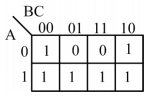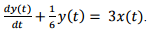# GATE 2016 Answer Key (Electrical): 7th February

Q. 1 – Q. 25 carry one mark each.

Q.1 The output expression for the Karnaugh map shown below is(A) A+ B'
(B) A+ C'
(C) A'+C'
(D) A'+C

Q.2 The circuit shown below is an example of a(A) low pass filter.
(B) band pass filter.
(C) high pass filter.
(D) notch filter.

Q.3 The following figure shows the connection of an ideal transformer with primary to secondary turns ratio of 1:100. The applied primary voltage is 100 V (rms), 50 Hz, AC. The rms value of the current I, in ampere, is __________.Q.4 Consider a causal LTI system characterized by differential equationThe response of the system to the input.
where u(t) denotes the unit step function, is

Answer:Q.5 Suppose the maximum frequency in a band-limited signal X(t) is 5 kHz. Then, the maximum frequency in X(t) cos(2000πt), in kHz, is ________.

Please report if you find any errors in the questions.
Answers/Solution of remaining questions are under evaluation and the same will be published here soon.
Keep watching the space